# Lecture 3 - Thermal Radiation & Intensity (1/19/99)Light --- | --- Mechanics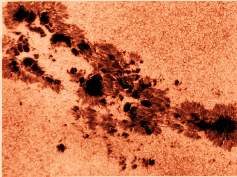Chapter 8-1, 8-6 (ZG4)

Notes:

pages 8 - 12

The region around a sunspot. The sunspot appears darker because it is cooler than the surrounding photosphere, a property of its blackbody spectrum. (Courtesy National Solar Observatory/Sacramento Peak)
Key Question: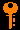## Investigations:

1. Brightness, Intensity and Flux
• What is flux and flux density?
• How is flux related to luminosity?
• How does the flux fall off with distance from a (point) source? Why?
• What are the units of intensity?
• What is a solid angle, and what are its units?
• How does the intensity of a uniform screen vary with distance? Why?
• How does the intensity from the surface of a uniformly lit flat surface vary with inclination angle?
• What area do we need to use in the intensity?
• How are flux and intensity related?
• Why do we include a factor of cosine(theta) in the formula?
• How do we get the 1/r^2 dependence of the flux from the constant intensity?
• What do we get if we integrate over the spectrum?
• Why do we integrate intensity over the hemisphere to get flux?
• How does the wavelength of the maximum brightness depend upon the temperature (Wien's Law)?
• How do we determine the surface temperature of the Sun?
• How does the surface flux emitted by a hot body depend upon the temperature (Stefan-Boltzmann Law)?
• Why did Planck introduce quantization of light into the blackbody formulation?
• What is a blackbody?
• What is Planck's blackbody function?
• Why does it matter whether you use B_nu or B_lambda?
• What is the integral of B_nu over the spectrum?
3. Thermal Properties of Gases
• What is the Maxwell-Boltzmann distribution of velocities?
• What is the root-mean-square (rms) velocity of a gas at temperature T?
• How is Boltzmann's constant k related to thermal energy in a gas?
• What is the rms velocity of Nitrogen (N2) at 300 K room temperature?
• What is a Boltzmann factor and why does it appear in all these different equations?
• What is the ideal gas low, and why do we use it in this form?
• What is number density and how is it related to mass density?

It is well-known that if you heat stuff up, it glows! If you turn the burner on your electric stove to high, it starts to glow red. A flame is bright, and burning coals glow red. If you've ever seen iron or steel being heated in a furnace, you know that as you heat it to higher and higher temperatures it glows red, yellow, bluish, then white-hot, and at the same time brighter and brighter.

There is obviously a connection with temperature and light emission. The hotter something is, the brighter it glows, and the "bluer" a color the light that it emits is.

The temperature of a body is a measure of how fast the atoms and molecules in it are jiggling about. Higher temperature means higher average velocity (actually, average squared-velocity). In physics, the temperature is measured using a scale called the Kelvin scale (K). This is the same degrees in the centigrade or Celsius scale (C) (a change of 1 K = 1 C), but measured from absolute zero (zero velocity, 0 K = -273 C = -460 F) instead of from the freezing of water ( 0 Celsius = 273 Kelvin = 32 F). Thus, water boils at 373 K (100 C = 212 F).

The kinetic energy of a particle is proportional to the square of the velocity:

E = m v^2 / 2

If you as what the distribution of kinetic energies of atoms in a gas at a temperature T is, you find a distribution that has is peaked at a characteristic kinetic energy, with few atoms at low energies (velocities), and few atoms at high energies (velocities). The average kinetic energy (we denote an average some quantity X by < X >):

< E > = < mv^2/2 > = 3kT/2

where k is Boltzmann's constant (k=8.6 x 10^-5 eV/K).

Because the electric force is transmitted by photons, then it turns out that whenever you apply a force to an electron, and it is accelerated, it will emit a photon. The fact that accelerating electrons always emit radiation is an important one in astonomy, and we will return to it more than once. At this point, it is important in that collisions between atoms can cause the electrons in the outer shells to accelerate, and thus emit radiation. The spectrum of this "thermal" radiation depends on the thermal distribution of velocities and thus kinetic energies of the atoms in the material.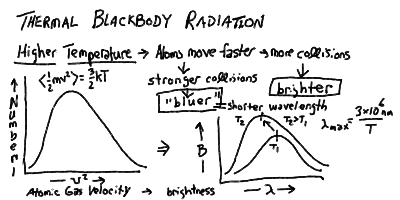If a material is opaque, that is if nearly all the photons emitted by an atom inside the matter will be absorbed and re-emitted by other atoms in the substance before escaping out through the surface, then the energy of the radiation will come to equilibrium with the energy in the thermal motions of the agitated electrons. Thus, opaque matter at a temperature T (in Kelvin) radiates a continuous spectrum, whose peak shift to shorter wavelength and total energy rise with the temperature: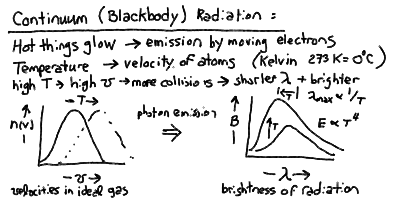The peak wavelength of the blackbody radiation is inversely proportional to the temperature, and the total power radiated per square meter of surface is proportional to the fourth power of the temperature: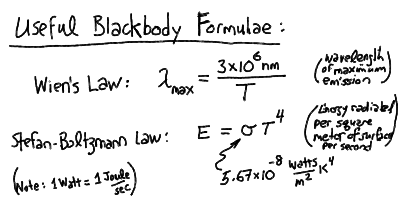Thus, we can relate the observed spectra and radiative fluxes to the temperature of a blackbody. Most objects (stars, planets, etc) have an approximately blackbody spectrum. Therefore, this is one of the most important concepts in astrophysics.

## Example Problem: Room Temperature

An example of the use of thermal equilibrium with blackbody radiation is the radiation from our own bodies at approximately 300 K!

First we calculate the surface flux of blackbody radiation:

Fsurf =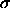T4 = 5.67 x 10-8 · ( 300 )4 = 459 W m-2

It appears we light up like a bunch of 100 Watt light bulbs! Actually, we are in near thermal equilibrium with our surroundings, except for our internal heat (only 50 W or so); our body regulates the heat flow with our thermal surroundings.

We can calculate the wavelength of the peak blackbody emission using Wien's Law: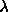max 2.898 x 10-3 m / (T/1K)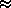3 x 10-3 m / 300 = 10-5 m = 10 µm

and lies in the mid-infrared (IR) part of the spectrum. This is why infrared goggles or infrared sensitive film can see a warm human body against a cold background in the dark!

Finally, we calculate the rms velocity of gas molecules in our lungs and around our bodies:

vrms = [ 3 k T / m ]1/2 = [ (3 · 1.3 x 10-23 · 300) / (28 · 1.67 x 10-27) ]1/2 = 515 m/s

The gas molecules are zipping around at over 500 m/s, even though the typical bulk wind speed is only 1 m/s! However, this is all in equilibrium with the atmosphere around us.

## Thermal Radiation and Gases - Some Web Resources

• If you heat stuff up, it glows and emits light. The hotter you make it, the brighter it gets, and the bluer in color it gets until it is "white hot" (which is of course hotter then "red hot"). This phenomenon is called thermal radiation.
• Thermal radiation is also called blackbody radiation because it is most easily calculated for a "black" substance that absorbs all light that falls on it (and also emits back the same light but in thermal equilibrium, and thus possibly at a different wavelength as we will see). Any opaque material will emit thermal blackbody radiation - that is photons with the characteristic thermal distribution.
• The thermal spectrum of photons is continuous, a smooth function of wavelength, in contrast to the spiky line emission at the discrete energy levels of atoms.
• The thermal spectrum is a maximum (brightest) at a characteristic wavelength L_max inversely proportional to the temperature:

Lmax = 2.89 mm ( 1 K / T )

• The total energy flux emitted in thermal radiation per square meter of surface of the body is proportional to the fourth power of the temperature:

E = S T4

where S is the Stefan-Boltzmann constant

S = 5.67 x 10-8 W m-2 K-4Prev Lecture ---Next Lecture ---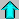Astr12 Index ---Astr12 Home

smyers@nrao.edu Steven T. Myers